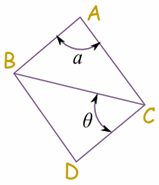# Angles

An angle measures the amount of turn

## Names of Angles

### As the Angle Increases, the Name Changes:Type of Angle Description
Acute Angle is less than 90°
Right Angle is 90° exactly
Obtuse Angle is greater than 90° but
less than 180°
Straight Angle is 180° exactly
Reflex Angle is greater than 180°
Full Rotation is 360° exactly

Try It Yourself:

### In One Diagram

This diagram might make it easier to remember:

Also: Acute, Obtuse and Reflex are in alphabetical order.Also: the letter "A" has an acute angle.

## Be Careful What You MeasureThe smaller angle is an Obtuse Angle, but the larger angle is a Reflex Angle

So when naming the angles make sure that you know which angle is being asked for!

## Positive and Negative Angles

When measuring from a line:

• a positive angle goes counterclockwise (opposite direction that clocks go)
• a negative angle goes clockwise### Example: −67°## Parts of an Angle

The corner point of an angle is called the vertex

And the two straight sides are called arms

The angle is the amount of turn between each arm.

## How to Label AnglesThere are two main ways to label angles:

1. give the angle a name, usually a lower-case letter like a or b, or sometimes a Greek letter like α (alpha) or θ (theta)

2. or by the three letters on the shape that define the angle, with the middle letter being where the angle actually is (its vertex).

Example angle "a" is "BAC", and angle "θ" is "BCD"